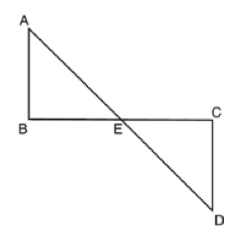### Prove: Triangle ABE is congruent to Triangle CDEClick or touch to draw on the diagram
Parallel lines form equal alternate interior angles
Vertical angles are equal
AD bisects BC at E; AB is parallel to CD
angle AEB is congruent to angle CED
BE is congruent to CE
ASA (Angle-Side-Angle)
angle B is congruent to angle C
Triangle ABE is congruent to Triangle CDE
Given
Definition